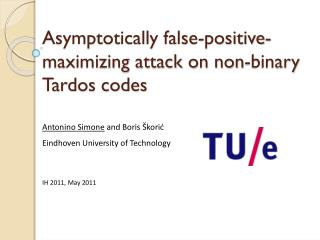Download PresentationAsymptotically false-positive-maximizing attack on non-binary Tardos codes

# Asymptotically false-positive-maximizing attack on non-binary Tardos codes - PowerPoint PPT PresentationDownload Presentation## Asymptotically false-positive-maximizing attack on non-binary Tardos codes

- - - - - - - - - - - - - - - - - - - - - - - - - - - E N D - - - - - - - - - - - - - - - - - - - - - - - - - - -
##### Presentation Transcript

1. Asymptotically false-positive-maximizing attack on non-binary Tardos codes Antonino Simone and Boris Škorić Eindhoven University of Technology IH 2011, May 2011

2. Outline • Forensic watermarking • Collusion attacks • q-aryTardos scheme • New parameterization of attack strategy • Accusation-minimizing attack • Performance of the Tardos scheme • False accusation probability • Results & Summary

3. originalcontent originalcontent content withhidden payload payload WM secrets payload WM secrets Detector Embedder Forensic Watermarking ATTACK Payload = some secret code indentifying the recipient

4. Collusion attacks "Coalition of pirates" Symbols received by pirates “Restricted Digit Model” Symbols allowed

5. Aim Trace at least one pirate from detected watermark BUT Resist large coalition  longer code Low probability of innocent accusation (FP) (critical!)  longer code Low probability of missing all pirates (FN) (not critical)  longer code AND Limited bandwidth available for watermarking code

6. q-aryTardos scheme (2008) m content segments biases Symbol biases drawn from distribution F embedded symbols • Arbitrary alphabet size q • Dirichletdistribution F • Symbol-symmetric n users c pirates Symbols allowed watermark after attack

7. Tardos scheme (cont.) • Accusation: • Every user gets a score • User is accused if score > threshold • Sum of scores per content segment • Given that pirates create y in segment i: • Symbol-symmetric p g0(p) g1(p) p

8. Accusation probabilities m = code length c = #pirates μ̃= expected coalition score per segment Pirates want to minimize μ̃ and make the innocent tail longer threshold • Curve shapes depend on: • F, g0, g1 (fixed ‘a priori’) • Code length • # pirates • Pirate strategy guilty innocent total score (scaled) Method to compute innocent curve [Simone+Škorić 2010] Big m  innocent curve goes to Gaussian

9. New parameterization of attack strategy Symbol-symmetric  only symbol occurrences matter Notation: α = #α in segment c pirates   α α = c For every segment: New attack parameterization that does not refer to symbols:

10. New parameterization of attack strategy (cont.) Due to the marking assumption, K0=0 and Kc=1 Kb can be pre-computed  faster computation Thanks to the new parameterization, we can write Which strategy minimizes μ̃?

11. μ̃-minimizing attack For each , the attack outputs the symbol y s. t. its occurrence value yminimizes T(b) (i. e. T(y)T() for each )

12. T(b) analysis Strong influence of  parameter More interesting case: Majority voting Minority voting

13. Results Gaussian approximation 

14. Results (cont.) Gaussian approximation 

15. Summary Results: • simple decoder accusation method in the Restricted Digit Model • new parameterization of the attack strategy • μ̃-minimizing attack is the strongest attack in asymptotic regime • not optimal attack for small coalitions •  parameter has a strong effect • For q>2 code length becomes better than for q=2, but only if c is large enough! • The larger q is, the larger c must be to obtain a code shorter than the case q=2 Thank you for your attention!